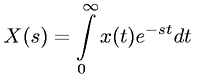Equations > Signal Processing > Laplace Transform Properties > Laplace transform definition

### Laplace transform definitionLatex Code:

MathML Code:

 $X\left(s\right)=\underset{0}{\overset{\infty }{\int }}x\left(t\right){e}^{-\mathrm{st}}\mathrm{dt}$

MathType 5.0: